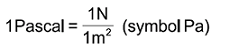## Units Of Measure

By Admin on Fri 10 February 2017

Commonly used physical and technical units symbols.

In the international System (SI) the physical and technical units are validated as follows.
Unit of length: Meter - (symbol m) Unit of Mass: Kilogram - (symbol Kg) Unit of time: Second - (symbol s) Unit of electrical current: Ampere - (symbol A) Unit of temperature: Kelvin - (symbol K) Unit of luminosity: Candle - (symbol CD)
Pressure. Old measuring units: Kilo-pond per Cm2: Kp/Cm2 Meter of water column: mH2O Millimetre of mercury column: mm-Hg Metric Atmosphere: at Atmosphere: ATM They were replaced in the SI from Pascal. One pascal corresponds to the pressure of 1 Newton, which is acting on the area of 1 m2.Unit Pa is a very low value and for standard industrial applications, the Bar (symbol bar) is used. 1 bar = 0.1 Mega-pascal (symbol Mpa = 1.000.000 Pa). The conversion from the old unit of measure to the new one (SI0 is the following: 1 Kp/Cm2 = 0.981 bar 1 bar = 1.02 Kp/Cm2 The conversion in the SI unit is also possible where the metric system is not yet used. Conversion: 1 bar = 14.50 psi 1 psi = 0.07 bar = 7.000 PaPressure values, except specific references, are referred to the atmospheric pressure.